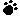Please review your test results by moving cursor to answer sheet icon.1.A)
B)
C)
D)

Your answer=, Correct answer=,

2.What is the difference between a histogram and a pareto chart?

A) A histogram is a less accurate version of a pareto chart.
B) A histogram is a type of pareto chart.
C) A pareto chart is a type of histogram.
D) A histogram and a pareto chart simply different terms for the same technique.Your answer=B, Correct answer=, Work Sheet:

3.A management control point where scope, budget, actual cost and schedule are integrated and compared to earned value for performance measurement is called a:

A) Code of accounts
B) Control packages
C) Control account
D) Account PlanYour answer=B, Correct answer=, Work Sheet:

4.A)
B)
C)
D)

Your answer=, Correct answer=,

5.If you want to compress a project schedule, what are two of the recommended alternatives to consider?

A) Fast Tracking and Schedule Network Analysis
B) Crashing and Fast Tracking
C) Resource Leveling and What-If Scenario Analysis
D) Crashing and Schedule Network AnalysisYour answer=A, Correct answer=, Work Sheet: